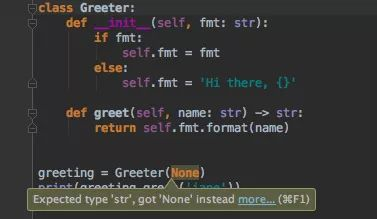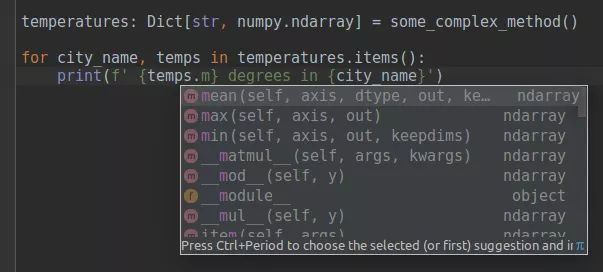# 愉快地迁移到 Python 3

（点击上方公众号，可快速关注）

http://python.jobbole.com/89031/

## 为数据科学家准备的 Python 3 特性指南

Python 已经成为机器学习和一些需处理大量数据的科学领域的主流语言。它支持了许多深度学习框架和其他已确立下来的数据处理和可视化的工具集。## 使用 pathlib 更好的对路径进行处理

pathlib 是 Python 3 中的默认模块，能帮你避免过多的使用 os.path.join：

from pathlib import Path

dataset = 'wiki_images'

datasets_root = Path('/path/to/datasets/')

train_path = datasets_root / dataset / 'train'

test_path = datasets_root / dataset / 'test'

for image_path in train_path.iterdir():

with image_path.open() as f: # note, open is a method of Path object

# do something with an image

p.exists()

p.is_dir()

p.parts

p.with_name('sibling.png') # only change the name, but keep the folder

p.with_suffix('.jpg') # only change the extension, but keep the folder and the name

p.chmod(mode)

p.rmdir()

pathlib 会节省你大量的时间，具体用法请参考 文档 和 说明

## 类型提示现在是 Python 的一部分啦

Pycharm 中类型提示示例：Python 已不再是一个小型的脚本语言了，如今的数据处理流程包含许多步骤，每步涉及不同的构架（而且有时会涉及不同的逻辑）

def repeat_each_entry(data):

""" Each entry in the data is doubled

<blah blah nobody reads the documentation till the end>

"""

index = numpy.repeat(numpy.arange(len(data)), 2)

return data[index]

repeat_each_entry(pandas.Series(data=[0, 1, 2], index=[3, 4, 5])) # returns Series with Nones inside

def repeat_each_entry(data: Union[numpy.ndarray, bcolz.carray]):

## 类型提示→运行中的类型检查

@enforce.runtime_validation

def foo(text: str) -> None:

print(text)

foo('Hi') # ok

foo(5)    # fails

@enforce.runtime_validation

def any2(x: List[bool]) -> bool:

return any(x)

any ([False, False, True, False]) # True

any2([False, False, True, False]) # True

any (['False']) # True

any2(['False']) # fails

any ([False, None, "", 0]) # False

any2([False, None, "", 0]) # fails

## 函数注释的其他惯例

# Python 3

from astropy import units as u

@u.quantity_input()

def frequency(speed: u.meter / u.s, wavelength: u.m) -> u.terahertz:

return speed / wavelength

frequency(speed=300_000 * u.km / u.s, wavelength=555 * u.nm)

# output: 540.5405405405404 THz, frequency of green visible light

## 使用 @ 进行矩阵乘积

# l2-regularized linear regression: || AX - b ||^2 + alpha * ||x||^2 -> min

# Python 2

X = np.linalg.inv(np.dot(A.T, A) + alpha * np.eye(A.shape)).dot(A.T.dot(b))

# Python 3

X = np.linalg.inv(A.T @ A + alpha * np.eye(A.shape)) @ (A.T @ b)

## 使用 ** 作通配符

import glob

# Python 2

found_images =

glob.glob('/path/*.jpg')

+ glob.glob('/path/*/*.jpg')

+ glob.glob('/path/*/*/*.jpg')

+ glob.glob('/path/*/*/*/*.jpg')

+ glob.glob('/path/*/*/*/*/*.jpg')

# Python 3

found_images = glob.glob('/path/**/*.jpg', recursive=True)

# Python 3

found_images = pathlib.Path('/path/').glob('**/*.jpg')

## Print 现在是函数

• 简化使用文件描述符的语法：

print >>sys.stderr, "critical error"      # Python 2

print("critical error", file=sys.stderr)  # Python 3

• 无需 str.join 输出制表符：

# Python 3

print(*array, sep='t')

print(batch, epoch, loss, accuracy, time, sep='t')

• 改写或重定义 print 的输出

# Python 3

_print = print # store the original print function

def print(*args, **kargs):

pass  # do something useful, e.g. store output to some file

@contextlib.contextmanager

def replace_print():

import builtins

_print = print # saving old print function

# or use some other function here

builtins.print = lambda *args, **kwargs: _print('new printing', *args, **kwargs)

yield

builtins.print = _print

with replace_print():

<code here will invoke other print function>

• print 函数可参与列表理解和其他语言构建。

# Python 3

result = process(x) if is_valid(x) else print('invalid item: ', x)

## 数值中的下划线（千位分隔符）

PEP-515 在数值中引入下划线。在 Python 3 中，下划线可用于整数、浮点数、复数的位数进行分组，增强可视性。

# grouping decimal numbers by thousands

one_million = 1_000_000

# grouping bits into nibbles in a binary literal

flags = 0b_0011_1111_0100_1110

# same, for string conversions

flags = int('0b_1111_0000', 2)

## 使用 f-strings 简便可靠的进行格式化

# Python 2

print('{batch:3} {epoch:3} / {total_epochs:3}  accuracy: {acc_mean:0.4f}±{acc_std:0.4f} time: {avg_time:3.2f}'.format(

batch=batch, epoch=epoch, total_epochs=total_epochs,

acc_mean=numpy.mean(accuracies), acc_std=numpy.std(accuracies),

avg_time=time / len(data_batch)

))

# Python 2 (too error-prone during fast modifications, please avoid):

print('{:3} {:3} / {:3}  accuracy: {:0.4f}±{:0.4f} time: {:3.2f}'.format(

batch, epoch, total_epochs, numpy.mean(accuracies), numpy.std(accuracies),

time / len(data_batch)

))

120  12 / 300  accuracy: 0.8180±0.4649 time: 56.60

f-strings 全称为格式化字符串，引入到了 Python 3.6：

# Python 3.6+

print(f'{batch:3} {epoch:3} / {total_epochs:3}  accuracy: {numpy.mean(accuracies):0.4f}±{numpy.std(accuracies):0.4f} time: {time / len(data_batch):3.2f}')

query = f"INSERT INTO STATION VALUES (13, {city!r}, {state!r}, {latitude}, {longitude})"

## ‘真实除法’与‘整数除法’的明确区别

velocity = data['distance'] / data['time']

n_gifts = money // gift_price  # correct for int and float arguments

## 严格排序

# All these comparisons are illegal in Python 3

3 < '3'

2 < None

(3, 4) < (3, None)

(4, 5) < [4, 5]

# False in both Python 2 and Python 3

(4, 5) == [4, 5]

• 防止不同类型实例的偶然分类

sorted([2, '1', 3])  # invalid for Python 3, in Python 2 returns [2, 3, '1']

• 有助于指示处理原始数据时发生的问题
旁注：适当的检查 None（两种版本的 Python 均需要）

if a is not None:

pass

if a: # WRONG check for None

pass

## 自然语言处理（NLP）中的统一编码标准（Unicode）

s = '您好'

print(len(s))

print(s[:2])

• Python 2: `6n��`

• Python 3: `2n您好`.

x = u'со'

x += 'co' # ok

x += 'со' # fail

Python 2 失效而 Python 3 如期输出（因为我在字符串中使用了俄文字母）

'a' < type < u'a'  # Python 2: True

'a' < u'a'         # Python 2: False

from collections import Counter

Counter('Möbelstück')

• Python 2 是：Counter({‘xc3’: 2, ‘b’: 1, ‘e’: 1, ‘c’: 1, ‘k’: 1, ‘M’: 1, ‘l’: 1, ‘s’: 1, ‘t’: 1, ‘xb6’: 1, ‘xbc’: 1})

• Python 3 是：Counter({‘M’: 1, ‘ö’: 1, ‘b’: 1, ‘e’: 1, ‘l’: 1, ‘s’: 1, ‘t’: 1, ‘ü’: 1, ‘c’: 1, ‘k’: 1})

## 字典和 **kwargs 的保存顺序

import json

x = {str(i):i for i in range(5)}

# Python 2

{u'1': 1, u'0': 0, u'3': 3, u'2': 2, u'4': 4}

# Python 3

{'0': 0, '1': 1, '2': 2, '3': 3, '4': 4}

from torch import nn

# Python 2

model = nn.Sequential(OrderedDict([

('conv1', nn.Conv2d(1,20,5)),

('relu1', nn.ReLU()),

('conv2', nn.Conv2d(20,64,5)),

('relu2', nn.ReLU())

]))

# Python 3.6+, how it *can* be done, not supported right now in pytorch

model = nn.Sequential(

conv1=nn.Conv2d(1,20,5),

relu1=nn.ReLU(),

conv2=nn.Conv2d(20,64,5),

relu2=nn.ReLU())

)

## 迭代拆封

# handy when amount of additional stored info may vary between experiments, but the same code can be used in all cases

# picking two last values from a sequence

*prev, next_to_last, last = values_history

# This also works with any iterables, so if you have a function that yields e.g. qualities,

# below is a simple way to take only last two values from a list

*prev, next_to_last, last = iter_train(args)

## 使用默认的 pickle 工具更好的压缩数组

# Python 2

import cPickle as pickle

import numpy

print len(pickle.dumps(numpy.random.normal(size=[1000, 1000])))

# result: 23691675

# Python 3

import pickle

import numpy

len(pickle.dumps(numpy.random.normal(size=[1000, 1000])))

# result: 8000162

## 更安全的解析

labels = <initial_value>

predictions = [model.predict(data) for data, labels in dataset]

# labels are overwritten in Python 2

# labels are not affected by comprehension in Python 3

## 超级简单的 super 函数

# Python 2

class MySubClass(MySuperClass):

def __init__(self, name, **options):

super(MySubClass, self).__init__(name='subclass', **options)

# Python 3

class MySubClass(MySuperClass):

def __init__(self, name, **options):

super().__init__(name='subclass', **options)

## 更好的 IDE：支持变量注释

• 以一种清晰的格式写出你的期望值

• 从 IDE 中得到很好的建议## 多重拆封

x = dict(a=1, b=2)

y = dict(b=3, d=4)

# Python 3.5+

z = {**x, **y}

# z = {'a': 1, 'b': 3, 'd': 4}, note that value for `b` is taken from the latter dict.

[*a, *b, *c] # list, concatenating

(*a, *b, *c) # tuple, concatenating

{*a, *b, *c} # set, union

Python 3.5+

do_something(**{**default_settings, **custom_settings})

# Also possible, this code also checks there is no intersection between keys of dictionaries

do_something(**first_args, **second_args)

## 永不过时的 API：使用仅带关键字的参数

model = sklearn.svm.SVC(2, 'poly', 2, 4, 0.5)

class SVC(BaseSVC):

def __init__(self, *, C=1.0, kernel='rbf', degree=3, gamma='auto', coef0=0.0, ... )

• 现在使用者必须明确输入参数名，比如：sklearn.svm.SVC(C=2, kernel=’poly’, degree=2, gamma=4, coef0=0.5)

• 这种机制将 API 的可靠性和灵活性进行了极好的融合

## 次重点：math 模块中的常量

# Python 3

math.inf # 'largest' number

math.nan # not a number

max_quality = -math.inf  # no more magic initial values!

for model in trained_models:

max_quality = max(max_quality, compute_quality(model, data))

## 次重点：单整型

Python 2 提供两种基本的整型：int 型（64 位有符整型）和用于长时计算的 long 型（C++ 后非常让人困惑）。

Python 3 有单精度的 int 型，它整合了长时计算的要求。

isinstance(x, numbers.Integral) # Python 2, the canonical way

isinstance(x, (long, int))      # Python 2

isinstance(x, int)              # Python 3, easier to remember

## 其他

• Enums 理论是有用处的，但：

• 在 python 的数据栈中，字符串输入已被广泛采用

• Enums 似乎并不与 numpy 交互，也不属于 pandas 范畴

• 协程听起来也很有希望做数据流程（参考 David Beazley 的幻灯片），但是我还没有看到他们被采用。

• Python 3 有稳定的 ABI

• Python 3 支持 unicode（因此 ω = Δφ / Δt 是可以的），但是最好还是使用虽旧但好用的 ASCII 码

• 一些库，比如：jupyterhub（云服务版 jupyter）、django 和新版 ipython，只支持 Python 3，因此一些听起来对你无用的特性却对那些你也许只想用一次的库非常有用。

### 数据科学特有的代码迁移难题（以及如何解决它们）

• 放弃支持嵌套参数

map(lambda x, (y, z): x, z, dict.items())

• 然而，它依然能很好的对不同的理解起效。

{x:z for x, (y, z) in d.items()}

通常来说，在 Python 2 和 Python 3 之间，理解也更好于‘翻译’。

• map()、.keys()、.values()、.items() 返回的是迭代器而不是列表。迭代器的主要问题是：

• 没有琐碎的分片

• 不能迭代两次

几乎全部的问题都可以通过将结果转化为列表来解决。

• 当遇到麻烦时，参见 Python 问答：我如何迁移到 Python 3

### 使用 python 教授机器学习和数据科学的主要问题

教授者应该首先花时间讲解什么是迭代器，它不能像字符串一样被分片、级联、倍乘、迭代两次（以及如何处理）。

我认为大部分教授者会很高兴规避这些细节，但是现在这几乎是不可能的。

# 结论

Python 2 与 Python 3 共存了将近 10 年，但是我们应当转移到 Python 3 了。

迁移到仅有 Python 3 的代码库后，研究所写的代码和产品开发的代码都会变得更简短、更可读、更安全。

现在大部分库同时支持这两个 Python 版本。我都有点等不及了，工具包放弃支持 Python 2 享受新语言特性的美好时刻快来吧。

迁移后代码绝对会更顺畅，参见“我们再也不要向后兼容啦！”

### 参考

• Key differences between Python 2.7 and Python 3.x

• Python FAQ: How do I port to Python 3?

• 10 awesome features of Python that you can’t use because you refuse to upgrade to Python 3

• Trust me, python 3.3 is better than 2.7 (video)

• Python 3 for scientists

看完本文有收获？请转发分享给更多人

关注「Python开发者」，提升Python技能